# Rationalizing the denominatorPage 1

#### WATCH ALL SLIDES

Slide 1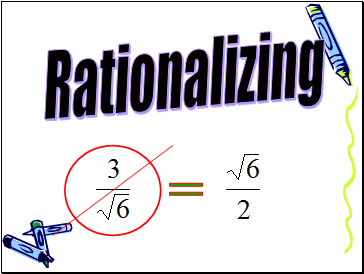## Rationalizing

Slide 2There is an agreement

in mathematics

that we don’t leave a radical

in the denominator

of a fraction.

Slide 3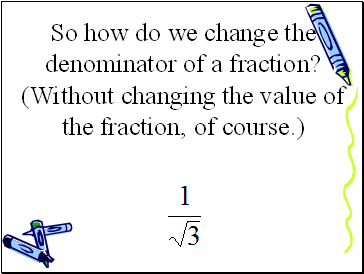So how do we change the denominator of a fraction?

(Without changing the value of the fraction, of course.)

Slide 4The same way we change the denominator of any fraction!

(Without changing the value of the fraction, of course.)

Slide 5We multiply the denominator

by the same number.

and the numerator

Slide 6By what number

to a rational number?

to change it

can we multiply

Slide 7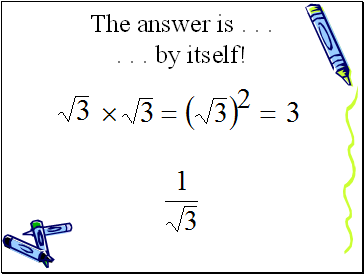The answer is . . .

. . . by itself!

Slide 8Remember,

is the number we square

to get n.

we’d better get n.

So when we square it,

Slide 9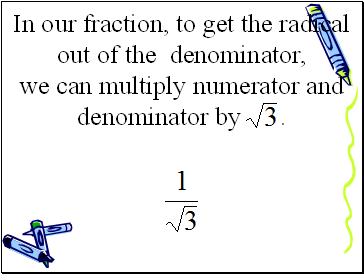Slide 10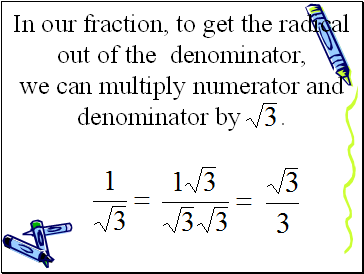Slide 11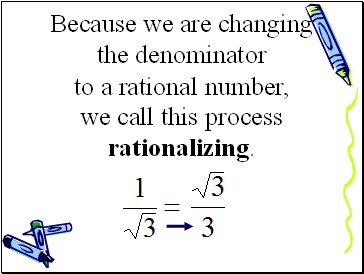Because we are changing the denominator

we call this process rationalizing.

to a rational number,

Slide 12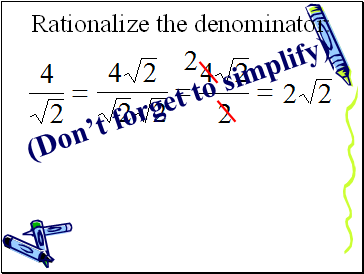Rationalize the denominator:

(Don’t forget to simplify)

Slide 13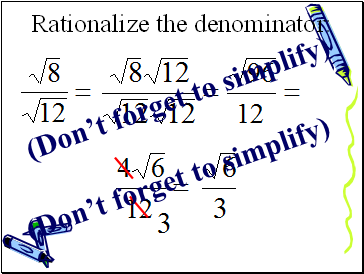Rationalize the denominator:

(Don’t forget to simplify)

(Don’t forget to simplify)

Slide 14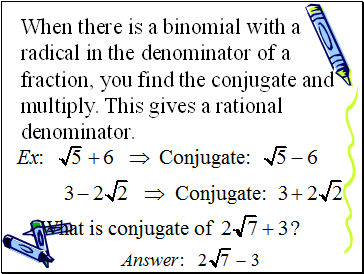When there is a binomial with a radical in the denominator of a fraction, you find the conjugate and multiply. This gives a rational denominator.

Slide 15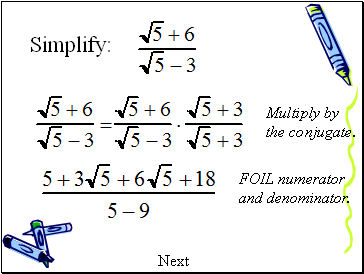Multiply by the conjugate.

FOIL numerator and denominator.

Next

Slide 16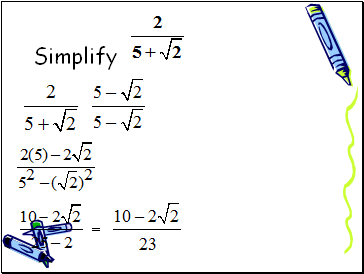Simplify

·

=

Slide 17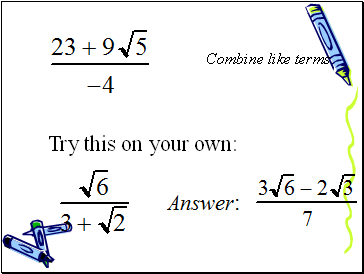Combine like terms